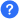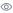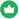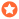In Scala programming language, scala.math package contains the various methods for performing basic numeric operations such as elementary exponential, logarithmic, root and trigonometric functions, etc.

Practice these Scala scala.math Package programs to learn the concept of performing mathematical operations, these programs contain the solved code, outputs, and the detailed explanation of the statements, functions used in the Scala scala.math Package programs.

### questions

Title
###### total questions: 11Questions

##### 11Views

##### 132